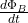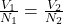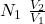## The 360-turn primary coil of a step-down transformer is connected to an ac line that is 120 V (rms). The secondary coil is to supply 15.0 A

Question

The 360-turn primary coil of a step-down transformer is connected to an ac line that is 120 V (rms). The secondary coil is to supply 15.0 A at 6.30 V (rms). 1) Assuming no power loss in the transformer, calculate the number of turns in the secondary coil. (Express your answer to two significant figures.)

in progress 0
2 weeks 2021-08-29T18:48:55+00:00 1 Answers 0 views 0

N₂ = 19 turns

Explanation:

A transform is a system with two different windings where the variation of the magnetic beam is the same, if there are no losses in the system we can use Faraday’s law

V₁ = -N₁v₂ = – N₂ \frac{d \Phi_B }{dt}in this case we look for the number of turns in the second winding

N₂2 =calculate us

N₂ = 360  6.30/ 120

N₂ = 18.9 turn

The number of turns must be an integer

N₂ = 19 turns